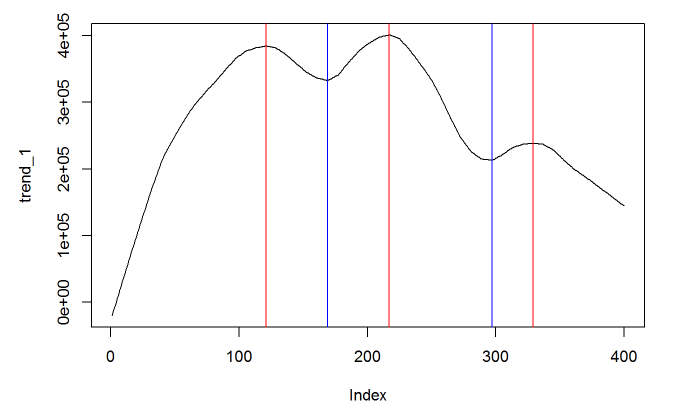[This article was first published on R - Data Science Heroes Blog, and kindly contributed to R-bloggers]. (You can report issue about the content on this page here)
Want to share your content on R-bloggers? click here if you have a blog, or here if you don't.### Introduction

Time series have maximum and minimum points as general patterns. Sometimes the noise present on it causes problems to spot general behavior.

In this post, we will smooth time series -reducing noise- to maximize the story that data has to tell us. And then, an easy formula will be applied to find and plot max/min points thus characterize data.

### What we have

```# reading data sources, 2 time series

# plotting...
plot(t1\$ts1, type = 'l')
plot(t2\$ts2, type = 'l')
```As you can see there are many peaks, but intuitively you can imagine a more smoother line crossing in the middle of the points. This can achieved by applying a Seasonal Trend Decomposition (STL).

### Smoothing the series

```# first create the time series object, with frequency = 50, and then apply the stl function.
stl_1=stl(ts(t1\$ts1, frequency=50), "periodic")
stl_2=stl(ts(t2\$ts2, frequency=50), "periodic")
```

Important: If you don’t know the `frequency` beforehand, play a little bit with this parameter until you find a result in which you are comfortable.

### Finding max and min

Creating the functions…

```ts_max<-function(signal)
{
points_max=which(diff(sign(diff(signal)))==-2)+1
return(points_max)
}

ts_min<-function(signal)
{
points_min=which(diff(sign(diff(-signal)))==-2)+1
return(points_min)
}
```

### Visualizing the results!

```trend_1=as.numeric(stl_1\$time.series[,2])

max_1=ts_max(trend_1)
min_1=ts_min(trend_1)

## Plotting final results
plot(trend_1, type = 'l')
abline(v=max_1, col="red")
abline(v=min_1, col="blue")
```With the line: `stl_1\$time.series[,2]` we are accessing the time series `trend` component. This is the smoothing method we will use, but there are others.

This first series has 3 maximums (red line) and 2 minimums (blue line) in the following places:

```# When the max points occurs:
max_1
``````# When the min points occurs:
min_1
```### Comparing two time series

```trend_2=as.numeric(stl_2\$time.series[,2])

max_2=ts_max(trend_2)
min_2=ts_min(trend_2)

# create two aligned plots
par(mfrow=c(2,1))

## Plotting series 1
plot(trend_1, type = 'l')
abline(v=max_1, col="red")
abline(v=min_1, col="blue")

## Plotting series 2
plot(trend_2, type = 'l')
abline(v=max_2, col="red")
abline(v=min_2, col="blue")
```Some conclusions from both plots:

• `Series 2` starts with a `min` while 1 does with a `max`
• `Series 1` has 3 `max` and 2 `min`, just the opposite to the other series

Why is this important? Because of the nature of the data, which is in next section.

### What is this data about?`ts1` and `ts2` are two typical responses to a brain stimulus, in other words: what happens with the brain when a person looks at a picture / move a finger / think in a particular thing, etc... Electroencephalography.

Some studies in neuroscience focus on averaging several responses to one stimulus -for example, to look at one particular picture. They present several times a particular image to the person. Averaging all of these signal/time series, you get the typical response.

Then you can predict based on the similarity between this typical response and the new image (stimulus) that the person is looking at.

#### Typical response (or Event Related Potential)It's important to get the when the positive peaks occur. In this case they are: `P1`, `P2` and `P3`. The same goes for the negative ones.

Note: It´s a common practice to invert negative and positive values.

#### Finally...

Typically the signal time length for this kind of studies last for 400ms, thus 1 point per millisecond, just the displayed plots. And the amplitude is in volts, (actually micro-volts). The same unit of measurement used by the notebook you are using now 😉###### that's all!DSH TwitterDSH FacebookMore DSH posts!